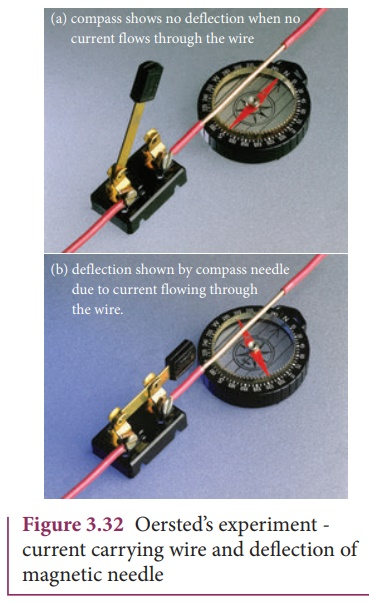Home | | Physics 12th Std | Magnetic Effects of Current

# Magnetic Effects of Current

Electric current passing through a wire deflects the nearby magnetic compass.

MAGNETIC EFFECTS OF CURRENT

## 1. Oersted experiment

In 1820, Hans Christian Oersted while preparing for his lecture in physics noticed that electric current passing through a wire deflects the nearby magnetic compass. By proper investigation, he observed that the deflection of magnetic compass is due to the change in magnetic field produced around current carrying conductor (Figure 3.32). When the direction of current is reversed, the magnetic compass deflects in opposite direction. This lead to the development of the theory ‘electromagnetism’ which unifies the two branches in physics, namely electricity and magnetism.## 2. Magnetic field arounda straight current carrying conductor and circular loop

### (a) Current carrying straight conductor:Suppose we keep a magnetic compass near a current carrying straight conductor, then the needle of the magnetic compass experiences a torque and deflects to align in the direction of the magnetic field at that point. Tracing out the direction shown by magnetic compass, we can draw the magnetic field lines at a distance. For a straight current carrying conductor, the nature of magnetic field is like concentric circles having their center at the axis of the conductor as shown in Figure 3.33 (a).

The direction of circular magnetic field lines will be clockwise or anticlockwise depending on the direction of current in the conductor. If the strength (or magnitude) of the current is increased then the density of the magnetic field will also increase. The strength of the magnetic field (B) decreases as the distance (r) from the conductor increases are shown in Figure 3.33 (b).

### b) Circular coil carrying currentSuppose we keep a magnetic compass near a current carrying circular conductor, then the needle of the magnetic compass experiences a torque and deflects to align in the direction of the magnetic field at that point. We can notice that at the points A and B in the vicinity of the coil, the magnetic field lines are circular. The magnetic field lines are nearly parallel to each other near the center of the loop, indicating that the field present near the center of the coil is almost uniform (Figure 3.34).

The strength of the magnetic field is increased if either the current in the coil or the number of turns or both are increased. The polarity (north pole or south pole) depends on the direction of current in the loop.

## 3. Right hand thumb rule

The right hand rule is a mnemonic to find the direction of magnetic field when the direction of current in a conductor is known.If we hold the current carrying conductor in our right hand such that the thumb points in the direction of current flow, then the fingers encircling the wire points in the direction of the magnetic field lines produced.The Figure 3.35 shows the right hand rule for current carrying straight conductor and circular coil.

## 4. Maxwell’s right handcork screw rule

This rule is used to determine the direction of the magnetic field. If we rotate a right-handed screw using a screw driver, then the direction of current is same as the direction in which screw advances and the direction of rotation of the screw gives the direction of the magnetic field. (Figure 3.36)### EXAMPLE 3.14

The magnetic field shown in the figure is due to the current carrying wire. In which direction does the current flow in the wire?.SolutionUsing right hand rule, current flows upwards.

Study Material, Lecturing Notes, Assignment, Reference, Wiki description explanation, brief detail
12th Physics : Magnetism and Magnetic Effects of Electric Current : Magnetic Effects of Current |